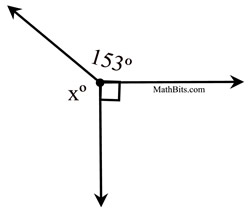Practice with Perpendicular Lines Terms of Use    Contact Person: Donna RobertsDirections: Read carefully and examine the diagrams!

1.
Given the diagram at the right with
lines m and n, and m∠1 = m∠2.
Which of the following statements is a conclusion which can be drawn?

Choose:
 ∠1 and ∠2 are right angles ∠1 and ∠2 are supplementary m ⊥ n All of the above.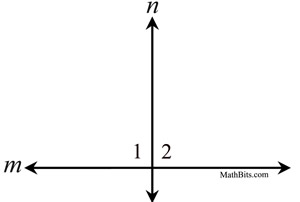2.
Given the diagram at the right, with
m
n . Which conclusion can be drawn regarding ∠1 and ∠2 ?
Choose:
 m∠1 = m∠2 ∠1 and ∠2 are complementary ∠2 is a right angle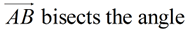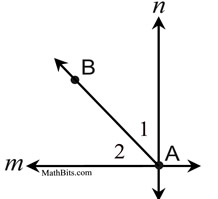3.
Given a b and the angles as labeled. Find the value of x.

Choose:
 2 6 10 12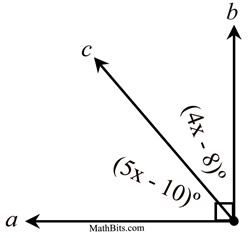4.
Given straight lines a and b.

a) Find the value of x.
Choose:
 21 42 48 52

b) Find the value of y.
Choose:
 92 142 132 138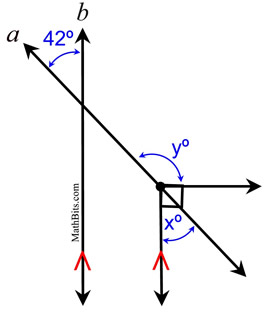5.
Given straight lines m and n.
Find the value of x.

Choose:
 21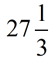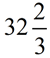54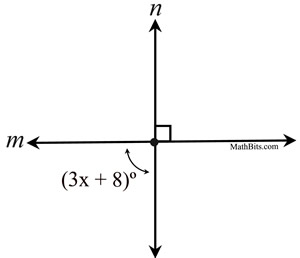6.
Given the diagram at the right.
Find the value of x
.
Choose:

 12 23 24 33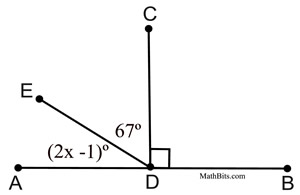7.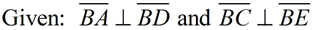.
If ∠1, ∠2, and ∠3 are congruent angles, the measure of each angle is:

Choose:
 30º 33º 45º 60º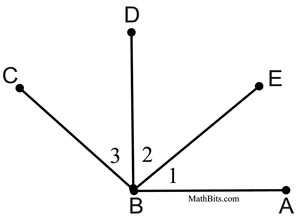8.
Given the diagram at the right as marked.
Find the measures of angles 1, 2, 3, 4 and 5.
 m∠1 Answer: 58º m∠2 Answer: 32º m∠3 Answer: 32º m∠4 Answer: 58º m∠5 Answer: 32º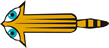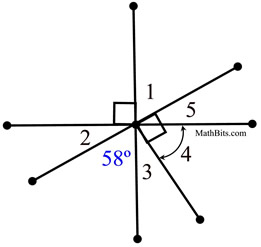9.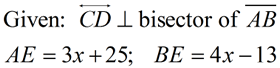Find AB.

Choose:

 76 139 278 417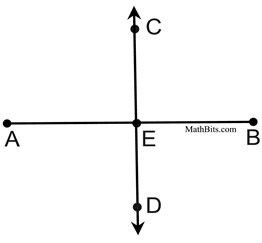10.
Given the diagram at the right as marked.
Find the value of x.

Choose:
 153 135 120 117